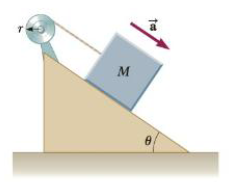Chapter 8, Problem 78AP

Chapter
Section
Textbook Problem

An object of mass M = 12.0 kg is attached to a cord that is wrapped around a wheel of radius r = 10.0 cm (Fig. P8.78). The acceleration of the object down the friction-less incline is measured to be a = 2.00 m/s2 and the incline makes ail angle θ = 37.0° with the horizontal. Assuming the axle of the wheel to be frictionless, determine (a) the tension in the rope, (b) the moment of inertia of the wheel, and (c) the angular speed of the wheel 2.00 s alter it begins rotating, starting from rest.Figure P8.78

(a)

To determine
The tension in the rope.

Explanation

Given info: The mass of the object is 12.0kg , acceleration due to gravity is 9.80m/s2 , the angle of the incline is 37.0° , and the tangential acceleration is 2.00m/s2 .

Explanation: The tension in the rope is given by MgsinθTMat=0 and it can be modified as T=M(gsinθat) .

The following diagram shows the free body diagram of block.

The formula for the tension in the rope is,

T=M(gsinθat)

• M is mass of the object.
• g is acceleration due to gravity.
• θ is angle of incline.
• at is tangential acceleration of the pulley

(b)

To determine
The moment of inertia of the wheel.

(c)

To determine
The angular speed of the wheel after the 2.00 s.

Still sussing out bartleby?

Check out a sample textbook solution.

See a sample solution

The Solution to Your Study Problems

Bartleby provides explanations to thousands of textbook problems written by our experts, many with advanced degrees!

Get Started

Find more solutions based on key concepts